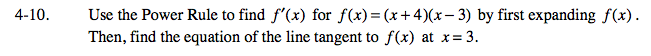### Home > CALC > Chapter 4 > Lesson 4.1.1 > Problem4-10

4-10.In order to find the equation of the tangent line at x = 3, you will need to find a point on the line and the slope at x =3.

To find a point, evaluate f(3)=_________

To find the slope, evaluate f '(3)= ________

Put it all together in point-slope form: yy1 = m(xx1)